# Formulas for GeometryPage 1

#### WATCH ALL SLIDES

Slide 1## Formulas for Geometry

Mr. Ryan

Slide 2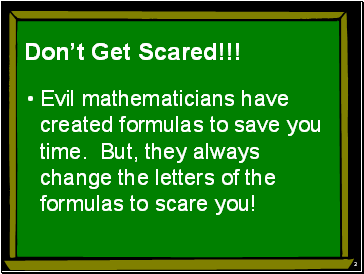2

Don’t Get Scared!!!

Evil mathematicians have created formulas to save you time. But, they always change the letters of the formulas to scare you!

Slide 33

## Perimeter

Any shape’s “perimeter” is the outside of the shape…like a fence around a yard.

Slide 44

Perimeter

To calculate the perimeter of any shape, just add up “each” line segment of the “fence”.

Slide 5Free powerpoint template: www.brainybetty.com

5

Perimeter

Triangles have 3 sides…add up each sides length.

8

8

8

8+8+8=24

The Perimeter is 24

Slide 66

Perimeter

A square has 4 sides of a fence

12

12

12

12

12+12+12+12=48

Slide 7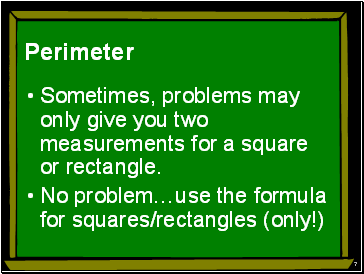7

Perimeter

Sometimes, problems may only give you two measurements for a square or rectangle.

No problem…use the formula for squares/rectangles (only!)

Slide 88

Remember

Squares ALL sides are equal…so if they give you one side, you know ALL the sides

Length=the Largest side

If they “leave” numbers out, they are equal to their opposite side. If they give you the bottom of a square/rectangle type shape then the top is the same

Slide 9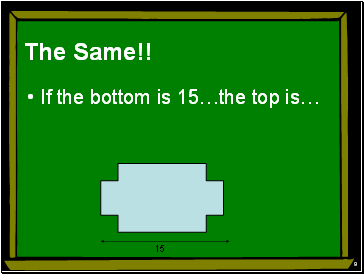9

The Same!!

If the bottom is 15…the top is…

15

Slide 1010

## Square/Rectangle Formula

Perimeter=2(Length+Width)

P= 2(25+14) P=2(20+20)

P= 50+28 P=40+40

P= 78 P=80

14

25

20

Slide 11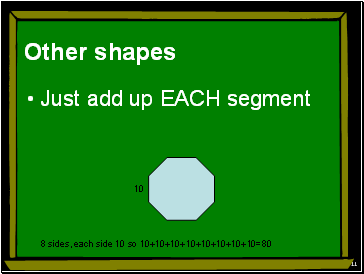11

## Other shapes

10

8 sides, each side 10 so 10+10+10+10+10+10+10+10=80

Slide 12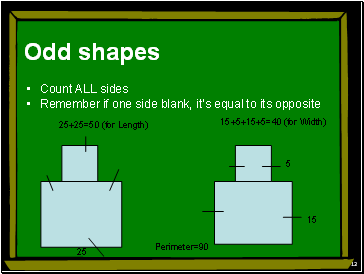12

## Odd shapes

Count ALL sides

Remember if one side blank, it’s equal to its opposite

25

15

5

25+25=50 (for Length)

15+5+15+5=40 (for Width)

Perimeter=90

Slide 13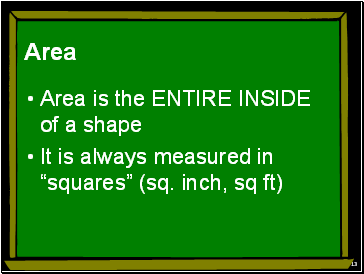Go to page:
1  2  3